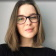# Can someone multiply 4 ( 2 x 3/4)

﻿
1. Sure.

First of all, the functions that are within parenthesis are supposed to be performed first, so let’s do that part first:

To multiply a whole number by a fraction, you must first multiply the numerator by the whole number, then divide that result by the denominator. However, since you have to then multiply that result by 4, we’ll not reduce the result until we’re done:

2 x 3/4 = 6/4

Now, multiply that fraction by 4:

4 × 6/4 = 24/4

Now reduce this by dividing the numerator by the denominator:

24/4 = 6

1. Mathematical expressions cannot always be expressed in a character string form without ambiguity. I am going first to make some assumptions and use the “*” sign where I am assuming that a multiplication is taking place:
4*[2*3/4]

Another issue is that the slash does not define accurately where a division is taking place. The issue can be resolved by using it only with one element above. This would not make a difference in this case, but consider the following expression:
2/3 * 4
This could be understood either as “[2/3] * 4” or as “2/[3 * 4]”, which are too different things.

In your case, we can write:
4 *{2*3*[1/4]}

This is a multiplication with four factors. The rules of multiplication allow us to change the order of the factors and to choose the order of the multiplications:
4 *[1/4]*2*3
{4 *[1/4]}*2*3

The expression in braces computes to 1, so we get:
1*2*3
The rest should be easy.

Psst... Stuck with your assignment? 😱Erica
OnlinePsst... Stuck with your assignment? 😱
Do you need an essay to be done?
Yes
Other assignmentWhat type of assignment 📝 do you need?
• Article review
• Case study
• Coursework
• Report
• Research Paper
• Other AssignmentHow many pages (words) do you need? Let's see if we can help you!